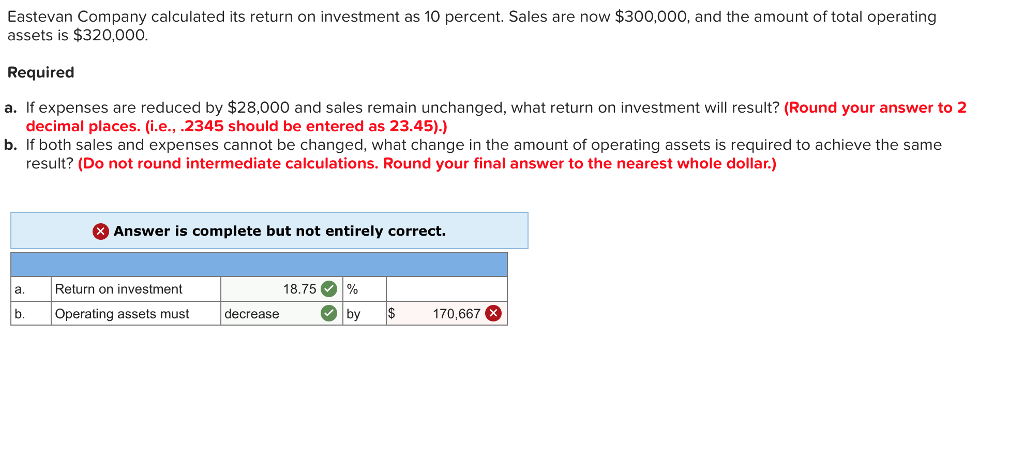# Question & Answer: Eastevan Company calculated its return on investment as 10 percent. Sales are now \$300,000, and the amount of total op…..Eastevan Company calculated its return on investment as 10 percent. Sales are now \$300,000, and the amount of total operating assets is \$320,000. Required a. If expenses are reduced by \$28,000 and sales remain unchanged, what return on investment will result? (Round your answer to 2 decimal places. (i.e., 2345 should be entered as 23.45).) b. If both sales and expenses cannot be changed, what change in the amount of operating assets is required to achieve the same result? (Do not round intermediate calculations. Round your final answer to the nearest whole dollar.)